Question
A diffraction grating with 13400 lines per 2.54 cm (i.e., per inch) is used to view the visible spectrum from hydrogen gas excited by an electrical discharge. At whatangle from the beam axis will the first order peak for the red photons (wavelength 656 nm) occur?

Please show all the work, it is much appreciated!

The number of lines on the grating 13400 lines/2.54 cm

The wavelength of the light = 656 nm

The slit width is d = 2.54/13400/cm = 1.895*10^-6 m

We have d*sin(θ) = mλ

sin(theta) = 656*10^-9 m/ 1.895*10^-6 m

theta = 20.24 degree

#### Earn Coins

Coins can be redeemed for fabulous gifts.

Similar Homework Help Questions
• ### A diffraction grating with 230 lines per mm is used in an experiment to study the...

A diffraction grating with 230 lines per mm is used in an experiment to study the visible spectrum of a gas discharge tube. At what angle from the beam axis will the first order peak occur if the tube emits light with wavelength of 421.2 nm? At what angle will the second order peak occur?

• ### ANGLE OF FIRST AND SECOND ORDER MAXIMA IN DIFFRACTION PATTERN!

A diffraction grating with 280 lines per mm is used in an experiment to study the visible spectrum of a gas discharge tube.1)At what angle from the beam axis will the first order peak occur if the tube emits light with wavelength of 585.5 nm?2)At what angle will the second order peak occur?

• ### (a) White light is spread out into its spectral components by a diffraction grating. If the...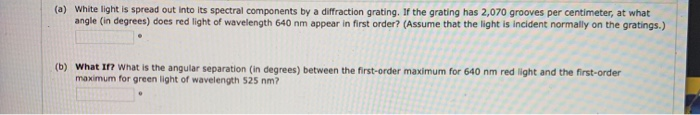(a) White light is spread out into its spectral components by a diffraction grating. If the grating has 2,070 grooves per centimeter, at what angle (in degrees) does red light of wavelength 640 nm appear in first order? (Assume that the light is incident normally on the gratings.) (b) What If? What is the angular separation (in degrees) between the first-order maximum for 640 nm red light and the first-order maximum for green light of wavelength 525 nm? 11. (-/1...

• ### ASAP PLEASE The spacing of ruled lines on a diffraction grating is 1,845 nm. The grating...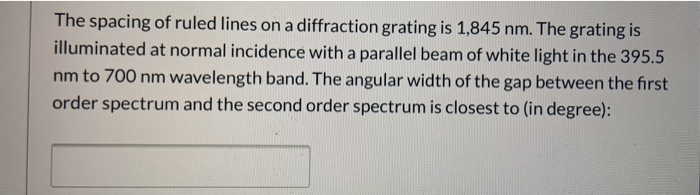ASAP PLEASE The spacing of ruled lines on a diffraction grating is 1,845 nm. The grating is illuminated at normal incidence with a parallel beam of white light in the 395.5 nm to 700 nm wavelength band. The angular width of the gap between the first order spectrum and the second order spectrum is closest to (in degree):

• ### diffraction grating

For your science fair project you need to design a diffractiongrating that will disperse the visible spectrum (400-700nm) over 30 degrees in first order.How many lines per millimeter does your grating need?What is the first-order diffraction angle of light from asodium lamp (wavelength = 589nm)?

• ### Intense white light is incident on a diffraction grating that has 460 lines/mm. (a) What is...

Intense white light is incident on a diffraction grating that has 460 lines/mm. (a) What is the highest order in which the complete visible spectrum can be seen with this grating? (Enter 1 for first order, 2 for second order, etc.) (b) What is the angular separation between the violet edge (400 nm) and the red edge (700 nm) of the first order spectrum produced by the grating?

• ### 3. (10 points) In a particular diffraction grating pattern, the red component (700 nm) in the...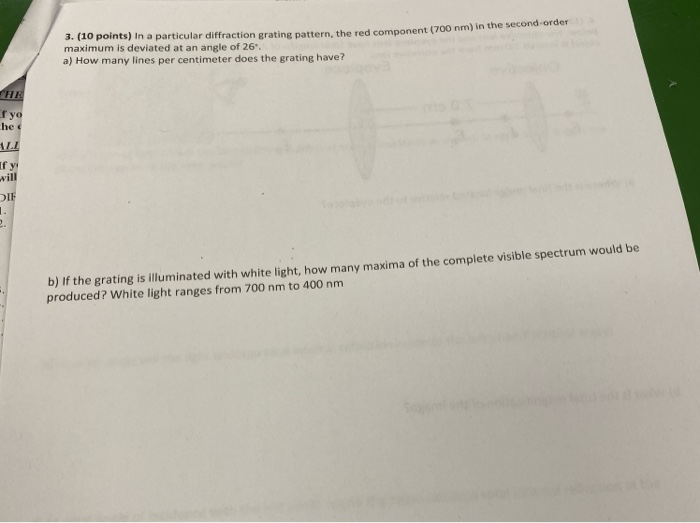3. (10 points) In a particular diffraction grating pattern, the red component (700 nm) in the second-order maximum is deviated at an angle of 26. a) How many lines per centimeter does the grating have? HE r yo he Г у will DIF deoslgby b) If the grating is illuminated with white light, how many maxima of the complete visible spectrum would be produced? White light ranges from 700 nm to 400 nm

• ### White light is spread out into its spectral components by a diffraction grating

White light is spread out into its spectral components by a diffraction grating. If the grating has 2000 lines per centimeter, at what angle does red light of wavelength 640 nm appear in first-order spectrum? (Assume that the light is incident normally on the grating.) Unpolarized light passes through two Polaroid sheets. The transmission axis of the analyzer makes an angle of 43.4° with the axis of the polarizer. (a) What fraction of the original unpolarized light is transmitted through the analyzer?...

• ### 28. When a narrow beam of white light is normally incident on a diffraction grating, you observe ...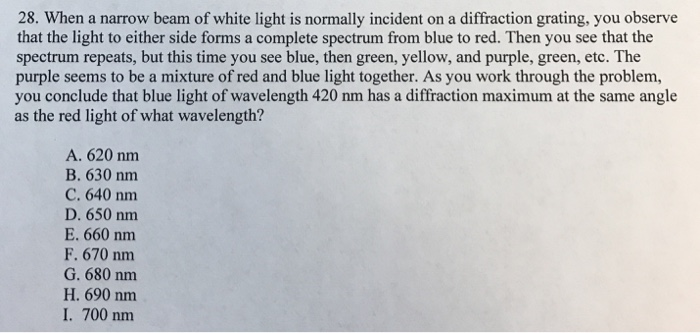28. When a narrow beam of white light is normally incident on a diffraction grating, you observe that the light to either side forms a complete spectrum from blue to red. Then you see that the spectrum repeats, but this time you see blue, then green, yellow, and purple, green, etc. The purple seems to be a mixture of red and blue light together. As you work through the problem, you conclude that blue light of wavelength 420 nm has...

• ### m) in the second-order 3. (10 points) in a particular diffraction grating pattern the red component...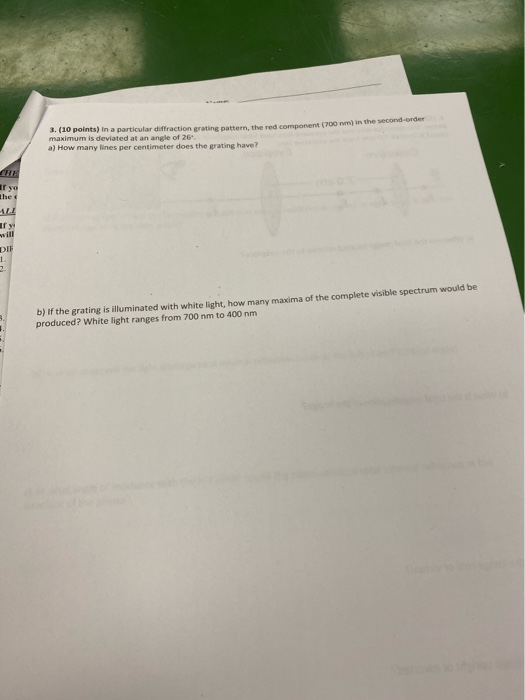m) in the second-order 3. (10 points) in a particular diffraction grating pattern the red component (700 maximum is deviated at an angle of 26 a) How many lines per centimeter does the rating have? If yo hed fy DIE - b) If the grating is illuminated with white light, how many maxima of the complete visible spectrum would be produced? White light ranges from 700 nm to 400 nm